## Example Questions

### Example Question #1 : Complex Fractions

Solve: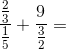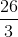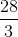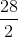Explanation:

To simplify a complex fraction, simply invert the denomenator and multiply by the numerator:Multiplying the numerator by the reciprocal of the denominator for each term we get: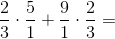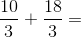Since we have a common denominator we can now add these two terms.### Example Question #2 : Complex Fractions

Simplify: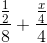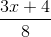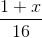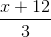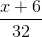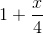Explanation:

Although you could look for the common denominator of the fraction as it has been written, it is probably easiest to rewrite the fraction in slightly simpler terms. Thus, recall that you can rewrite your fraction as: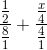Using the rule for dividing fractions, you can rewrite your expression as: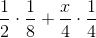Then, you can multiply each set of fractions, getting: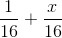This makes things very easy, for then your value is:### Example Question #3 : Complex Fractions

Simplify: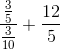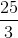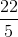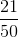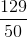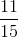Explanation:

For this problem, begin by rewriting the complex fraction, using the rule for dividing fractions: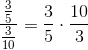This is much easier to work on. Cancel out thes and theand the, this gives you: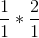, which is merely.  Thus, your problem is: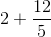The common denominator is, so you can rewrite this as: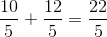### Example Question #4 : Complex Fractions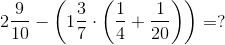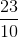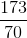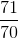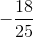Explanation:

Begin by simplifying all terms inside the parentheses. Begin with the innermost set. Find a common denominator for the two terms. In this case, the common denominator will be twenty: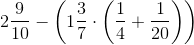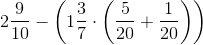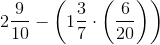Simplify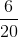to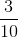and convert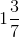to not a mixed fraction: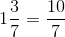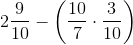Multiply the two fractions in the parentheses by multiplying straight across (A quick shortcut would be to factor out the 10 on top and bottom).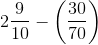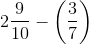Now convert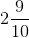to a non-mixed fraction. It will become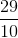.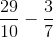In order to subtract the two fractions, find a common denominator. In this case, it will be 70.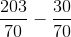Now subtract, and find the answer!is the answer